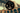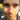### Lanky Dan Blog# Rust for Java Devs - Creating Variables (first Rust post)

January 13, 2018

rustjava

I’m going to do something different today and write about Rust instead of Java. This is something that I want to give a try and if it all goes well I will continue writing some posts about Rust in the future.

I will be writing about learning Rust from the perspective of a Java developer by making comparisons where appropriate to help you (and me) understand what is going on. These will most likely be shorter posts and honestly might not even contain that much information, but hopefully someone finds these sort of posts helpful and makes it all worth it.

A good resource for learning Rust is the Rust Book which I am using to teach myself the language and I will keep mentioning it whenever I can because I have found it very helpful.

This post will focus on creating variables.

The first thing to mention is that Rust variables are immutable by default whereas Java variables are mutable by default.

Therefore if you tried to compile the Rust code below.

``````let x = 1;
x = 2;``````

You will get the following compiler error.

``````error[E0384]: re-assignment of immutable variable `x`
--> src\main.rs:3:3
|
2 |   let x = 1;
|       - first assignment to `x`
3 |   x = 2;
|   ^^^^^ re-assignment of immutable variable``````

The error message is very clear in telling us what has gone wrong. We have altered the value of `x` which is currently immutable and thus lead to the program blowing up.

If you compared this to the very similar Java code.

``````int x = 1;
x = 2;``````

This compiles without any issues.

Although if we ran the code below instead.

``````final int x = 1;
x = 2;``````

This does not compile and is the equivalent of the earlier Rust code.

If we wanted to have mutable variables in Rust we need to do a bit more coding, not much though, and add the `mut` keyword to the variable declaration. Just like how in Java we need to add `final` to make a variable immutable (which we did above).

Below is what the earlier failed example will look like with the addition of `mut`.

``````let mut x = 1;
x = 2;``````

Compiling this will cause no errors.

Something I am sure you noticed from the earlier examples when coming from writing Java is that the type of the variable in Rust does not need to be included. The compiler is able to figure out it’s type from the value it has been given and from then on the type cannot change. This is not something that can currently be done in Java as you are required to declare the type of all variables when created. Below is what the earlier example would look like if we included the type when creating the variable.

``````let mut x: u32 = 1;
x = 2;``````

Here we have declared that `x` is of type `u32` (unsigned integer). This is required when the type cannot be figured out by the compiler due to many possible types being returned by a function, but for the above example it is not necessary.

Next up we have the concept called Shadowing. Lets look at an example first.

``````let x = 1;
let x = x + 1;
let x = x + 2;
println!("Value of x: {}", x);``````

I personally think this looks a bit weird and if you tried to do something like that in Java it would not work, as seen below.

``````int x = 1;
int x = x + 1;
int x = x + 2;``````

Outputs the compiler error.

``````Exception in thread "main" java.lang.Error: Unresolved compilation problems:
Duplicate local variable x
Duplicate local variable x``````

Back to looking at he Rust code. What happens is that each `let x` that occurs after the original will steal the name of `x` but will still create a new variable behind the scenes. As you can see from the example even though we have used `x` on both sides the line it will still compile and uses the current value of `x` before creating a shadowed `x` with a new value.

By the way if you still wondering the value of `x` from the example is 4.

Shadowing also allows you to change the type of a value because shadowing is simply creating a new instance each time. Therefore the code below works fine even though the types have changed.

``````let x = 1;
let x = String::from("I'm not a number anymore");
println!("Value of x: {}", x);``````

Obviously this code is trivial and you wouldn’t just create variables and not use them like this but for an example its fine. The compiler will give you a warning though for not making use of the original `x`.

``````warning: unused variable: `x`
--> src\main.rs:2:7
|
2 |   let x = 1;
|       ^
|``````

Although a type can be changed when shadowing a variable it cannot be done via `mut`. As this uses the same variable throughout it’s lifetime only it’s value can be modified but not it’s type. So if you tried to compile similar code to the above example using `mut` instead you will get a complier error.

``````let mut x = 1;
x = String::from("I'm not a number anymore");
println!("Value of x: {}", x);``````

Outputs the error.

``````error[E0308]: mismatched types
--> src\main.rs:3:7
|
3 |   x = String::from("I'm not a number anymore");
|       ^^^^^^^^^^^^^^^^^^^^^^^^^^^^^^^^^^^^^^^^ expected integral variable, found struct `std::string::String`
|
= note: expected type `{integer}`
found type `std::string::String`
= help: here are some functions which might fulfill your needs:
- .capacity()
- .len()``````

The error tells you that the original type of `x` was an integer (`u32`) but found another line that tried to set its value to `std::string::String` instead. The compiler also gives you suggestions of functions that might be useful incase you just forgot to type something or used the wrong function.

Finally lets look at constants. These are always immutable in Rust as they cannot have `mut` added to their declarations. They are defined using the `const` keyword instead of `let` and can be declared in any scope, including the global scope. They also must be set to a constant expression and therefore cannot be set equal to a function’s return value which is computed at runtime.

``const ONE_THOUSAND: u32 = 1000;``

The type of the constant must also be included and cannot be inferred from the constants value.

These are similar to using `static final` values in Java that are used for constant values that can be shared between methods and if made public can be used by other classes. Below is the equivalent of the above code but in this context is scoped to the class it is declared in.

``private static final int ONE_THOUSAND = 1000;``

That should do it for a first look into Rust variables. In conclusion we have looked into declaring variables in Rust while comparing them to their Java equivalents and highlighting the differences between the two. The main take away is that in Rust variables are immutable by default and their types can be inferred from their value whereas Java is mutable by default and types must always be declared.

Although this post is quite basic I hope it was somewhat useful, especially for Java developers looking to get started with Rust. As I mentioned earlier on, the Rust Book is worth having a look at if you want to give Rust a proper try.Written by Dan Newton.